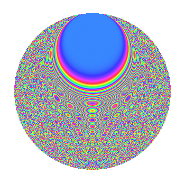# Properties

 Label 2015.2.cpLevel 2015 Weight 2 Character orbit cp Rep. character $$\chi_{2015}(32,\cdot)$$ Character field $$\Q(\zeta_{12})$$ Dimension 840 Sturm bound 448

# Related objects

## Defining parameters

 Level: $$N$$ = $$2015 = 5 \cdot 13 \cdot 31$$ Weight: $$k$$ = $$2$$ Character orbit: $$[\chi]$$ = 2015.cp (of order $$12$$ and degree $$4$$) Character conductor: $$\operatorname{cond}(\chi)$$ = $$65$$ Character field: $$\Q(\zeta_{12})$$ Sturm bound: $$448$$

## Dimensions

The following table gives the dimensions of various subspaces of $$M_{2}(2015, [\chi])$$.

Total New Old
Modular forms 912 840 72
Cusp forms 880 840 40
Eisenstein series 32 0 32

## Trace form

 $$840q + 4q^{2} - 420q^{4} + 4q^{5} - 24q^{8} + 24q^{9} + O(q^{10})$$ $$840q + 4q^{2} - 420q^{4} + 4q^{5} - 24q^{8} + 24q^{9} + 16q^{11} - 32q^{12} - 12q^{13} - 8q^{15} - 420q^{16} + 8q^{17} - 8q^{19} + 8q^{20} - 8q^{21} - 16q^{22} - 16q^{23} + 32q^{24} + 20q^{25} + 32q^{26} + 28q^{32} + 32q^{33} + 20q^{34} - 96q^{36} - 96q^{37} - 8q^{39} + 48q^{40} - 20q^{41} + 120q^{42} + 40q^{43} - 72q^{44} - 100q^{45} + 8q^{46} - 124q^{48} + 428q^{49} + 4q^{50} - 40q^{52} - 12q^{53} - 32q^{54} - 68q^{55} + 72q^{56} - 96q^{57} + 168q^{58} + 16q^{59} + 148q^{60} + 24q^{61} - 36q^{63} + 840q^{64} - 12q^{65} + 96q^{67} - 4q^{68} + 8q^{69} - 136q^{70} + 48q^{71} + 144q^{72} - 160q^{73} + 36q^{74} - 32q^{75} + 80q^{76} - 64q^{77} - 164q^{78} + 44q^{80} + 428q^{81} - 40q^{82} - 80q^{84} + 4q^{85} - 104q^{86} - 128q^{87} - 112q^{88} - 12q^{89} + 108q^{90} + 16q^{91} + 96q^{92} - 32q^{95} - 48q^{96} + 40q^{97} + 32q^{98} + 144q^{99} + O(q^{100})$$

## Decomposition of $$S_{2}^{\mathrm{new}}(2015, [\chi])$$ into irreducible Hecke orbits

The newforms in this space have not yet been added to the LMFDB.

## Decomposition of $$S_{2}^{\mathrm{old}}(2015, [\chi])$$ into lower level spaces

$$S_{2}^{\mathrm{old}}(2015, [\chi]) \cong$$ $$S_{2}^{\mathrm{new}}(65, [\chi])$$$$^{\oplus 2}$$